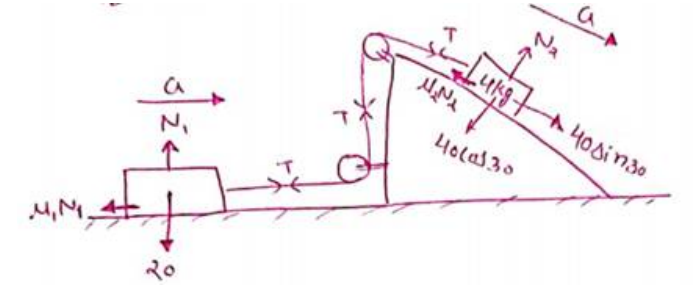# Solve the following :`
Question:

If the tension in the string in figure is $16 \mathrm{~N}$ and the acceleration of each block is $0.5 \mathrm{~m} / \mathrm{s}^{2}$, find the friction coefficients at the two contacts with the blocks.

Solution:For $2 \mathrm{Kg}$ block

$T-\mu_{1} N_{1}=2 \times a$

$16-\mu_{1}(20)=2 \times(0.5)$

$15=20 \mu_{1}$

$\mu_{1}=0.75$

For $4 \mathrm{Ka}$ block

$40 \sin 30-T-\mu_{2} N_{2}=4 a$$40 \sin 30-T-\mu_{2} N_{2}=4 a$

$40 \times\left(\frac{1}{2}\right)-T-\mu_{2}(40 \cos 30)=4 a$

$20-16-\mu_{2} \times 40 \times \frac{\sqrt{3}}{2}=4 \times 0.5$

$\mu_{2}=0.06$